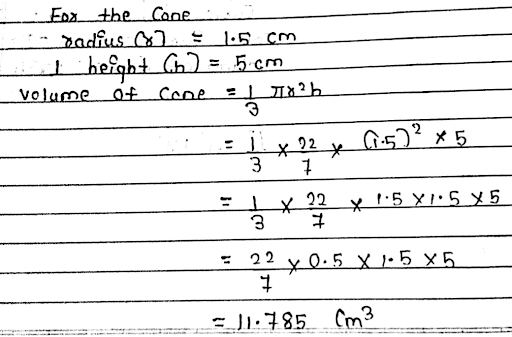Find the volume of a cone if the radius of its base is 1.5 cm and its perpendicular height is 5 cm.

Find the volume of a cone if the radius of its base is 1.5 cm and its perpendicular height is 5 cm.

verified

The correct Answer is 11.79 cm3=11.79 cm3